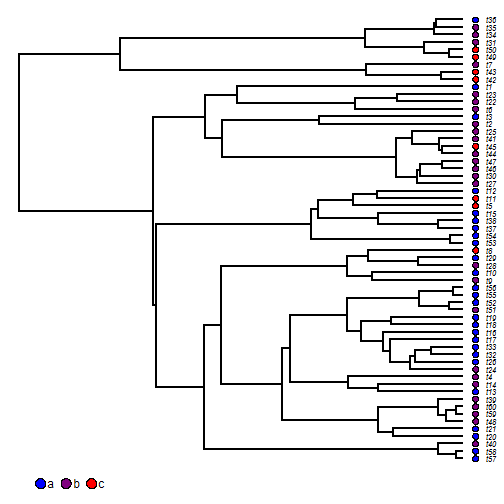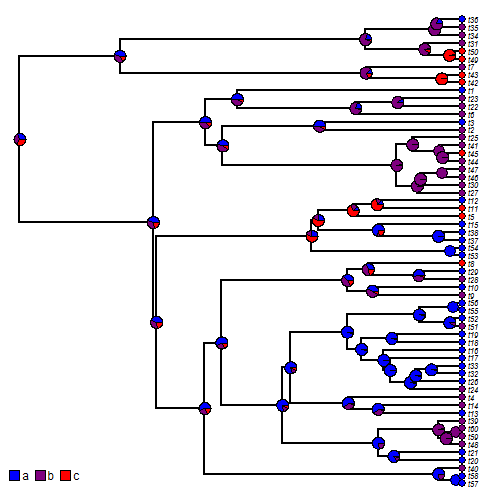## Tuesday, November 28, 2017

### Running `make.simmap` in parallel

Today a phytools user asked on my GitHub page if there was any way to permit `make.simmap` run in parallel.

Note that the issue motivating this report likely stems from a replacement of the method to do matrix exponentiation in the latest CRAN version of phytools. This has already been fixed - although, of course, if we are using `make.simmap` with large trees, complex models, or generating many samples, it will still be slow!

`make.simmap` does not have a parallelization option; however, for the basic method we are just estimating the transition matrix, Q, via Maximum Likelihood, and then sampling histories for the discrete character conditioned on this fitted model. Consequently, we ought to be able to first compute Q once and then run our sampler on multiple cores. In theory, this can be done using `parallel::mclapply`.

Unfortunately, parallelization is not supported in R for Windows. Nonetheless, it is possible to run `mclapply` which pseudo-parallelizes our analysis. This is so that functions & packages using parallelization don't break on Windows - but it will not run any faster!

Here is what that might look like. I have assumed we have 4 cores to play with.

``````library(phytools)
## our data:
cols<-setNames(colorRampPalette(c("blue","red"))(3),
c("a","b","c"))
dotTree(tree,x,fsize=0.7,ftype="i",colors=cols)
````````````## first fit our model
fit<-fitMk(tree,x,model="ARD")
fit
``````
``````## Object of class "fitMk".
##
## Fitted (or set) value of Q:
##           a         b         c
## a -0.667821  0.667821  0.000000
## b  0.337475 -0.715334  0.377859
## c  0.989418  0.070697 -1.060115
##
## Fitted (or set) value of pi:
##         a         b         c
## 0.3333333 0.3333333 0.3333333
##
## Log-likelihood: -51.296883
##
## Optimization method used was "nlminb"
``````
``````## extracted the fitted transition matrix:
fittedQ<-matrix(NA,length(fit\$states),length(fit\$states))
fittedQ[]<-c(0,fit\$rates)[fit\$index.matrix+1]
diag(fittedQ)<-0
diag(fittedQ)<--rowSums(fittedQ)
colnames(fittedQ)<-rownames(fittedQ)<-fit\$states
## ready to run our analysis
library(parallel)
trees<-mclapply(1:4,function(n,tree,x,fixedQ) make.simmap(tree,x,Q=fixedQ,nsim=100),
tree=tree,x=x,fixedQ=fittedQ,mc.cores=if(.Platform\$OS.type=="windows") 1L else 4L)
``````
``````## make.simmap is sampling character histories conditioned on the transition matrix
##
## Q =
##            a           b          c
## a -0.6678208  0.66782077  0.0000000
## b  0.3374750 -0.71533391  0.3778589
## c  0.9894179  0.07069738 -1.0601152
## (specified by the user);
## and (mean) root node prior probabilities
## pi =
##         a         b         c
## 0.3333333 0.3333333 0.3333333
``````
``````## Done.
``````
``````## make.simmap is sampling character histories conditioned on the transition matrix
##
## Q =
##            a           b          c
## a -0.6678208  0.66782077  0.0000000
## b  0.3374750 -0.71533391  0.3778589
## c  0.9894179  0.07069738 -1.0601152
## (specified by the user);
## and (mean) root node prior probabilities
## pi =
##         a         b         c
## 0.3333333 0.3333333 0.3333333
``````
``````## Done.
``````
``````## make.simmap is sampling character histories conditioned on the transition matrix
##
## Q =
##            a           b          c
## a -0.6678208  0.66782077  0.0000000
## b  0.3374750 -0.71533391  0.3778589
## c  0.9894179  0.07069738 -1.0601152
## (specified by the user);
## and (mean) root node prior probabilities
## pi =
##         a         b         c
## 0.3333333 0.3333333 0.3333333
``````
``````## Done.
``````
``````## make.simmap is sampling character histories conditioned on the transition matrix
##
## Q =
##            a           b          c
## a -0.6678208  0.66782077  0.0000000
## b  0.3374750 -0.71533391  0.3778589
## c  0.9894179  0.07069738 -1.0601152
## (specified by the user);
## and (mean) root node prior probabilities
## pi =
##         a         b         c
## 0.3333333 0.3333333 0.3333333
``````
``````## Done.
``````
``````trees
``````
``````## []
## 100 phylogenetic trees with mapped discrete characters
##
## []
## 100 phylogenetic trees with mapped discrete characters
##
## []
## 100 phylogenetic trees with mapped discrete characters
##
## []
## 100 phylogenetic trees with mapped discrete characters
``````

At the end, though, instead of one `"multiSimmap"` object, we have a list of 4! Fortunately, we can combine these in a fairly easy way as follows:

``````trees<-do.call(c,trees)
if(!("multiSimmap"%in%class(trees))) class(trees)<-c("multiSimmap",class(trees))
trees
``````
``````## 400 phylogenetic trees with mapped discrete characters
``````

Neat.

Now, let's compute a summary & visualize the results:

``````obj<-summary(trees)
obj
``````
``````## 400 trees with a mapped discrete character with states:
##  a, b, c
##
## trees have 43.7325 changes between states on average
##
## changes are of the following types:
##        a,b a,c   b,a   b,c    c,a  c,b
## x->y 18.29   0 7.945 8.745 8.1425 0.61
##
## mean total time spent in each state is:
##               a          b         c    total
## raw  27.2549076 23.1011251 8.2695555 58.62559
## prop  0.4648978  0.3940451 0.1410571  1.00000
``````
``````plot(obj,colors=cols,ftype="i",fsize=0.7)
x=0,y=2)
``````The tree & data for this example were simulated as follows:

``````tree<-pbtree(n=60)
Q<-matrix(c(-1,1,0,
1,-2,1,
0,1,-1),3,3)
rownames(Q)<-colnames(Q)<-letters[1:3]
x<-as.factor(sim.history(tree,Q)\$states)
``````

I would love to hear a report from someone working on a Unix-alike system (e.g., Mac OS) who can report if this indeed works on their machine!

1.2.